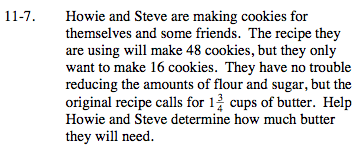### Home > MC2 > Chapter 11 > Lesson 11.1.1 > Problem11-7

11-7.$\text{Per 48 cookies there needs to be }1\frac{3}{4} \text{ cups of butter.}$

Set up a proportion.

$\frac{\text{48 cookies}}{1\frac{3}{4}\text { cups of butter}} = \frac{\text{16 cookies}}{x\text{ cups of butter}}$

Solve for x.

$\frac{7}{12}\text{ of a cup}$